RUSSIAN JOURNAL OF EARTH SCIENCES, VOL. 19, ES5003, doi:10.2205/2019ES000667, 2019

# Far fields of internal gravity waves from a source moving in the ocean with an arbitrary buoyancy frequency distribution

1Ishlinsky Institute for Problems in Mechanics RAS, Moscow, Russia

2Shirshov Institute of Oceanology RAS, Moscow, Russia

### Abstract

The generation of internal gravity waves in the ocean with an arbitrary distribution of the buoyancy frequency generated by a moving source of perturbations is considered. The basic dispersion characteristics determining the properties of the generated far wave fields are studied analytically and numerically. The results of numerical computations of internal wave fields for different generation modes are presented. It is shown that the far wave fields of a separate mode can be presented as a sum of wave trains. The article investigates the specific characteristics of how these wave trains are generated. The proposed approach can be used to model internal wave wakes from a moving typhoon.

### 1. Introduction

The moving atmospheric cyclones have a significant impact on the ocean circulation, the local sea surface temperature, and the generation of internal gravity waves. It is expected that the wave fields generated by this generation can play a significant role in various mechanisms of energy transfer in the ocean interior. The tangential wind stress induced by a moving hurricane forms a structure in the ocean in the form of a wave train or trace. The experimental detection of these wave structures was one of the impressive achievements of modern oceanology [Bulatov and Vladimirov, 2012, 2015; Gill, 1984; Massel, 2015; Mei et al., 2017; Morozov, 2018; Pedlosky, 2010; Sutherland, 2010; Velarde et al., 2018].

The propagation of internal dispersion waves in stratified ocean media has specific features related to the dependence of the propagation velocity on the wavelength. If a perturbation source moves in such a medium, then it creates a wave pattern around itself, the main features of which are the lines of the constant phase. The structure of wave patterns at large distances from the moving source (much greater than its dimensions) is practically independent of its shape and is mainly determined by the dispersion law and the source velocity [Bulatov and Vladimirov, 2012, 2015; Svirkunov and Kalashnik, 2014].

The system of hydrodynamic equations describing the wave perturbations is a complex mathematical problem, and the main results of solving the problems on the internal wave generation can be obtained only in the most general integral form or numerically. In numerical calculations, the ocean is usually represented by a simplified hydrodynamic system with a model density distribution. The integral representations of solutions require the development of asymptotic methods for their research. These methods allow us to conduct high-quality analysis and estimation of the solutions obtained in the field measurements of internal waves in the ocean [Frey et al., 2017; Furuichi et al., 2008; Gill, 1984; Kang and Fringer, 2010; Lecoanet et al., 2015; Morozov et al., 2003, 2008; Tiugin et al., 2012].

The modern approaches to the description of linear internal waves are based on the representation of wave fields by Fourier integrals, an analysis of their asymptotics, and the geometric construction of enveloping wave fronts in the framework of the kinematic theory of dispersion waves. Based on the kinematic theory, it is possible to formulate analytical representations only for the phase surfaces of wave fields of internal gravity waves from a moving typhoon [Svirkunov and Kalashnik, 2014].

The goal of this work is to solve a more complex problem of constructing asymptotics. We also describe special features of the phase and amplitude structures of the far fields of internal gravity waves generated by a localized perturbation source. The source is moving in the ocean of a finite depth with an arbitrary stratification. Indeed, at large distances, the real sources of perturbations (moving typhoon) allow a physically justified approximation by a system of localized point sources taken with certain weights [Bulatov and Vladimirov, 2018; Furuichi et al., 2008; Gill, 1984; Kang and Fringer, 2010; Lecoanet et al., 2015]. The use of real hydrology allows us to take into account the specific characteristics of wave dynamics with regard to the variability of the marine environment density observed in the field measurements of internal waves in the ocean.

### 2. The Problem Formulation and Analytic Representations of the Solutions

The elevation $\eta$ of the field of internal gravity waves generated by a source that begins to move at a velocity $V$ at a depth $z_0$ at a time $t=0$ in a stratified ocean of finite depth $- H < z < 0$ can be determined from the problem [Bulatov and Vladimirov, 2012, 2015, 2018]

\begin{equation} \tag*{(1)} L \eta = \Theta (t) Q (t, x, y, z, z_0) \end{equation} \begin{eqnarray*} L= \frac{\partial^2}{\partial t^2} (\frac{\partial^2}{\partial x^2} \frac{\partial^2}{\partial y^2} \frac{\partial^2}{\partial z^2}) + N^2(z) ( \frac{\partial^2}{\partial x^2} + \frac{\partial^2}{\partial y^2}) \end{eqnarray*}

where $\Theta (t) =0$, $t<0$, $\Theta(t) =1$, $t \geq 0$, $N^2(z)$ is the Brunt-Vaisala frequency and $Q (t, x, y, z, z_0)$ is the source density distribution. The form of the function $Q (t, x, y, z, z_0)$ depends on the source character. If we consider the force directed upwards along the vertical as a moving source, then we have

\begin{eqnarray*} Q (t, x, y, z, z_0) = \delta(z-z_0) (\frac{\partial^2 \delta(x-Vt)}{\partial x^2}\delta(y) + \end{eqnarray*} \begin{eqnarray*} \frac{\partial^2 \delta(y)}{\partial y^2}\delta(x-Vt)) \end{eqnarray*}

Further, we consider the case of a moving point source of a mass:

\begin{eqnarray*} Q (t, x, y, z) = \end{eqnarray*} \begin{eqnarray*} \frac{\partial^2 }{\partial t \partial z_0}(\delta (x-Vt) \delta(t) \delta(y) \delta (z-z_0)) \end{eqnarray*}

Since the problem under study is linear, the obtained solutions can further be used to obtain representations for the fields of internal waves generated by nonlocal sources of a different nature [Bulatov and Vladimirov, 2012, 2015; Svirkunov and Kalashnik, 2014].

The boundary conditions are taken in the following form (the vertical axis $z$ is directed upwards)

Then the solution of problem (1)–(2) that describes the steady-state wave mode of far wave fields in the coordinate system moving together with the source has the form of a sum of wave modes:

\begin{eqnarray*} \eta (\xi, y, z) = \sum \limits_n \eta_n (\xi, y, z) \end{eqnarray*}

where

\begin{eqnarray*} \eta_n = \frac{1}{4\pi} \int\limits_{-\infty}^{\infty} D_n(z, z_0, \nu) \times \end{eqnarray*}

\begin{equation} \tag*{(3)} \exp(i(\mu_n(\nu) \xi - \nu y)) d \nu \end{equation} \begin{eqnarray*} D_n(z, z_0, \nu)= \frac{\mu_n^2 (\nu)}{\mu_n^2 (\nu) + \nu^2} \times \end{eqnarray*} \begin{eqnarray*} \left( \frac{\mu_n (\nu)}{\nu} \frac{\partial \mu_n (\nu)}{\partial \nu} +1 \right) \times \end{eqnarray*} \begin{eqnarray*} f_n (z, \nu) \frac{\partial f_n (z_0, \nu)}{\partial z_0}, \qquad \xi= x- Vt \end{eqnarray*}

The eigenfunctions $f_n (z, \nu)$ and the eigenvalues $\mu_n^2(\nu)$ are determined from the problem [Bulatov and Vladimirov, 2012, 2015, 2018]

\begin{eqnarray*} \frac{\partial ^2 f_n (z, \nu)} {\partial z^2}+ (\mu_n^2 (\nu) + \nu^2) \times \end{eqnarray*} \begin{eqnarray*} \left( \frac{N^2(z)}{\mu_n^2 (\nu) V^2} -1 \right) f_n (z, \nu) =0 \end{eqnarray*} \begin{eqnarray*} f_n (0, \nu) = f_n (-H, \nu) =0 \end{eqnarray*}

For an arbitrary distribution of the buoyancy frequency, this spectral problem is solved numerically. The dispersion dependence $\mu_n(\nu)$ is a solution of the equation

\begin{eqnarray*} \omega_n^2 (k) = V^2 \mu_n^2 ( \nu), \; k^2 = \mu_n^2 ( \nu) + \nu^2 \end{eqnarray*}

where $\omega_n (k)$ is an eigenvalue of the basic vertical spectral problem of internal gravity waves [Pedlosky, 2010; Sutherland, 2010; Velarde et al., 2018]. In what follows, we consider a separate wave mode, and the subscript $n$ is omitted.

The integrals (3) describe the field of a separate mode of internal gravity waves far from the trajectory of motion of a local perturbation source for large $\xi$ and $y$. The asymptotics of these integrals can be calculated by the stationary phase method under the assumption that, for example, $\xi$ is a large parameter and the ratio $y/\xi$ is fixed. The function $\eta(\xi, y, z)$ is an even function of $y$, and therefore, for definiteness, we can assume that $y>0$. The stationary points of the phase function of integral (3) are determined by solving the equation

\begin{equation} \tag*{(4)} \mu'(\nu) = y/\xi \end{equation}

We denote $q= \max \mu'(\nu)$. Then for $\xi < y/q$, the phase function of integral (3) has no stationary points, and the field $\eta(\xi, y, z)$ is exponentially small. For $\xi> y/q$, the phase function has several stationary points (at least two, $\nu_{1,2} = \pm \nu^*$) which appear in pairs $\nu_{{1,2}_k} = \pm \nu^*_k$, $k= 1, 2, \ldots, K$, because the function $\mu_n (\nu)$ is odd ($\nu_k^*$ are positive roots of (4)). Then the asymptotics of a separate wave mode at a far distance from the perturbation source has the form

\begin{equation} \tag*{(5)} \eta \approx \sum\limits_{k=1}^{K} \frac{D(z, z_0, \nu_k^*)}{\sqrt{2\pi \xi | \mu"(\nu_k^*)}} \cos (\mu(\nu_k^*) \xi - \nu_k^*y - \frac{\pi}{4}) \end{equation}

where the sum is taken over all of the $K$ stationary points. The wave region is concentrated inside the wedge with the half-angle $\varphi =\arctan q$. On the ray $y/\xi =$const, the field decreases as $\sqrt{\xi}$, and in this case, the wave length $\lambda$ of the wave mode along the ray is constant: $\lambda= 2 \pi /(\mu(\nu^*) - \nu^* y/\xi)$. Solving the equation $\mu (\nu) - \nu y /\xi = \Phi$ together with (4) for $\xi$ and $y$, we obtain the lines of equal phase determined parametrically (with parameter $\nu$)

\begin{eqnarray*} \xi = \frac{\Phi}{ \mu ( \nu) - \mu' ( \nu)\nu} \end{eqnarray*} \begin{eqnarray*} y = \frac{\mu' ( \nu) \Phi}{ \mu ( \nu) - \mu' ( \nu)\nu} \end{eqnarray*}

Asymptotics (5) cease to work near the wave fronts, i.e., in the case where the stationary points tend to reach for each other and $\mu" (\nu) \to 0$. We consider the asymptotics in a neighborhood of the wave fronts, assuming that $\xi$ and $y$ are large. The wave fronts are determined by the values $\nu_i^0$ for which $\mu"(\nu_i^0) =0$. Then in the neighborhood of the points $\nu = \nu_i^0$, the function $\mu(\nu)$ admits the expansion

\begin{eqnarray*} \mu ( \nu) = \mu ( \nu_i^0) + q_i(\nu-\nu_i^0) - b_i(\nu-\nu_i^0)^3 + \ldots \end{eqnarray*}

and the position of the wave fronts is determined by the formula $y= q_i\xi$. The asymptotics of the wave field near each of the wave fronts have the form [Bulatov and Vladimirov, 2012, 2015]

\begin{equation} \tag*{(6)} \eta_i \approx \frac{D(z, z_0, \nu_i^0)}{\sqrt{3 b_i \xi}} Ai \frac{\xi q_i - y}{\sqrt{3 b_i \xi}} \end{equation}

Here,

\begin{eqnarray*} Ai (\tau) = \frac{1}{2 \pi} \int\limits_{-\infty}^{\infty} \cos (\tau t - t^3/3) dt \end{eqnarray*}

is the Airy function. The total wave field is obtained by summing over all values $\nu_i^0 (i=1, \ldots, I)$ for which $\mu"(\nu_i^0) =0$; $\eta= \sum_{i=1}^I \eta_i$.

### 3. Numerical Results and Discussions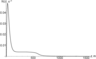Figure 1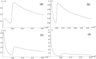Figure 2

The numerical computations are based on the use of the typical distribution of the Brent-Vaisaala frequency with a single thermocline maximum, which is shown in Figure 1 [Massel, 2015; Mei et al., 2017; Morozov, 2018; Pedlosky, 2010 Sutherland, 2010; Velarde et al., 2018]. All numerical results are further given for the second wave mode and the values $z= 40$ m, $z_0= 60$ m. We introduce the notation: $M= V/C$, where $C= \partial \omega (k)/ \partial k$ is the maximal value of the group velocity of internal waves of the second mode which is equal to $C= 0.836$ m/s. Figure 2a,b,c,d illustrate the results of computations of the function $\mu'(\nu)$ for different fixed values of $M$. These results show that, for this buoyancy frequency distribution, one can observe the dispersion pattern for which the function $\mu'(\nu)$ has three extrema (the value of $I$ is equal to 3): two maxima $q_1, \;q_2$ (left and right) and a minimum $q_3$, and always $q_3 < q_{1,2}$.

Numerical computations show that the qualitative pattern of dispersion dependence $\mu'(\nu)$ is strongly affected by the values of $M$. Indeed, for $M<1$, the values of the two maxima $q_{1,2}$ (left and right in Figure 2) are of the same order, for small values of $M$, the left maximum is less than the right one, as $M$ increases, the value of the left maximum of the function $\mu'(\nu)$ increases, and for $M_0 = 0.546 <1$, the values of the left and right maxima coincide: $q_1=q_2$ (this means that the two wave fronts simultaneously enter the fixed observation point). Thus, for $M< M_0$, we have $q_1 < q_2$, and for $M> M_0$, we have $q_1>q_2$. Further, as $M$ increases, the values of the left maximum $q_1$ of the function $\mu'(\nu)$ increase noticeably, and for $M>1$, this maximum is always attained for $\nu=0$.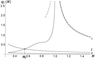Figure 3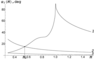Figure 4

For critical generation modes when the source velocity $V$ is close to the maximal group velocity of internal wave propagation $C$, i.e., as $M \to 1$, the maximal value $q_1$ of the function $\mu'(\nu)$ asymptotically behaves as $1/\sqrt{| M^2 -1|}$ [Bulatov and Vladimirov, 2012, 2015]. Figure 3 presents the maximal and minimal values of the function $\mu'(\nu)$ depending on the parameter $M$: $q_i = q_i (M), \; i= 1, \;2,\;3$. In this figure, the dashed line shows the function $1/\sqrt{| M^2 -1|}$ which, as numerical computations show, well describes the qualitative behavior of the dispersion dependence $\mu'(\nu)$ for critical modes of generation of internal waves for wide ranges of the perturbation source velocities. Figure 4 illustrates the dependence of the half-angles $\varphi_i(M) = \arctan q_i (M), \; i= 1, \;2,\;3$ of the corresponding wave fronts on the parameter $M$. The half-angles $\varphi_2(M)$, $\varphi_3(M)$ corresponding to the right maximum and minimum of the function $\mu'(\nu)$ are monotone decreasing functions of the parameter $M$. The half-angle $\varphi_1(M)$ corresponding to the left maximum $q_1$ of the function $\mu'(\nu)$ first increases to 90 degrees (critical generation mode $M\to 1$) and then monotonically decreases as the parameter $M> 1$ increases.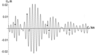Figure 5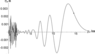Figure 11

Figure 5Figure 11 illustrate the results of numerical computations of the wave fields by formulas (3); the points on the graphs show the position of the corresponding wave fronts. The internal waves far field generated by a moving perturbation source (for $y, \: \xi \geq H$) is a sum of three wave trains. The times at which they come to a fixed point in the observation variable are determined by three wave fronts. For $M<1$, the far field of a separate mode within the internal gravity waves located at a far distance from the moving perturbation source at the observation point fixed with respect to behaves as follows. For $\xi < y/Q_1$ ($Q_1 = q_2$ for $M < M_0$, $Q_1 = q_1$ for $M > M_0$), the wave field is negligibly small. For $\xi=\xi_1=y/Q_1$, the wave front of the first wave train enters the fixed observation point, and the field in a neighborhood of the wave front is expressed in terms of the Airy function ($\eta=\eta_1$, formula (6)).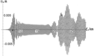Figure 6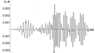Figure 8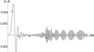Figure 9

For $y/Q_1 < \xi < y/Q_2$ ($Q_2 = q_1$ for $M < M_0$, $Q_2 = q_2$ for $M > M_0$), the field consists of a single wave train. For $\xi=\xi_2=y/Q_2$, the second wave train also enters the observation point, and the field in a neighborhood of its wave front is also expressed in terms of the Airy function, formula (6). For $y/Q_2 < \xi < y/q_3$ ($q_3$ is equal to the minimal value of $\mu'(\nu)$ for the given $M$), the wave field consists of two terms: $\eta = \eta_1 + \eta_2$. For $\xi = \xi_3 = y/q_3$, the third wave train also enters the observation point (formula (6)), and for $\xi>y/q_3$, the field consists of three terms: $\eta=\eta_1 + \eta_2 +\eta_3$. As numerical computations show, for $M < 1$, the wave trains that have amplitudes of the same order of magnitude and commensurable wave lengths make equal contributions to the total wave field, and therefore, the wave pattern is a complicated system of wave beatings (Figure 5, Figure 6). The contribution of the third term to the total wave field is noticeable at the distances $y$, $\xi \leq H$ and only for $MFigure 5). As numerical computations show, for this distribution of the buoyancy frequency, the contribution of the term$\eta_3$corresponding to the minimum of the function$\mu'(\nu)$to the far fields of generated internal waves (for$y$,$\xi \geq H$and for$M>M_0$) is negligibly small, and it does not practically make any contribution to the total field (Figure 8, Figure 9).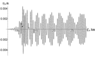Figure 7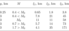Table 1 Indeed, for$M>M_0$, the typical values of$q_3$are small compared to the values of$q_{1,2}$, and hence the term$\eta_3$makes a contribution for large values of$\xi$for which the wave field amplitude (decreasing as$\sqrt{\xi}$for large$\xi$) is sufficiently small. For$M= M_0$, we have$q_1=q_2$, and hence there are two wave trains simultaneously entering the observation point fixed with respect to the variable$y$for$\xi = \xi_1 = \xi_2 = y/q_1 = y/q_2$(Figure 7). Table 1 shows the results of computations of the quantities$\xi_1, \xi_2, \xi_3$for the values$y, M$used in the numerical computations (Figure 5Figure 9). For$M>1$, the wave pattern of generated far fields is practically completely determined by the properties of the wave train corresponding to the left maximum$q_1$of the function$\mu'(\nu)$. This wave train is significantly greater in the amplitude and has a greater wave length (lower frequency), the wave trains corresponding to the right maximum$q_2$and the minimum$q_3$of the function$\mu'(\nu)$have lesser wave lengths (higher frequencies) and hence are significantly less in the amplitude. Therefore, no complicated wave beating pattern is observed for$M>1$, and the wave trains are significantly spread in the space and propagate independently of each other; namely, when one of the wave trains arrives, the contribution of another wave train to the total field becomes negligibly small. For$M>1$, the contribution to the wave field of the third term corresponding to the minimum$q_3$of the function$\mu'(\nu)$is small, and the total field is practically completely determined only by the behavior of two terms (Figure 9).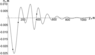Figure 10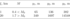Table 2 If the far fields of internal waves are considered at an observation point fixed with respect to the variable$\xi$, then the wave pattern varies with the increasing distance from the traverse of the source motion as follows (Figure 10, Figure 11). First, the wave front enters the observation point$y=y_1=q_3\xi$, and$y < q_3\xi$, the field is determined only by the term$\eta = \eta_3$. Further, as the traverse distance increases, the second wave front arrives for$y = y_2 = \xi Q_2$($Q_2 = q_1$for$M < M_0$and$Q_2 = q_2$for$M > M_0$). In the interval$\xi q_3 < y < \xi Q_2$, the total field is the sum of two terms:$\eta = \eta_2 + \eta_3$, and finally, at large distances from the traverse, the third wave front arrives for$y = y_3 = \xi Q_1$($Q_1 = q_2$for$M < M_0$and$Q_1 = q_1$for$M > M_0$). In the interval$\xi Q_2 < y < \xi Q_1$, the wave field is the sum of three terms:$\eta = \eta_1 + \eta_2 + \eta_3$. For$y > y_3$, the far wave field is exponentially small. Table 2 shows the results of computations of the quantities$y_1, y_2, y_3$for the values of$\xi, \: M$used in numerical computations (Figure 10, Figure 11). An important specific feature of the internal wave generation by a moving perturbation is that the characteristic velocity of the typhoon motion is 3–5 m/s, which is significantly greater than the typical maximal group velocity of internal waves in the ocean. Thus, when studying the formation of the ocean wave wake behind the moving typhoon, the most physically realistic case is the motion of a perturbation source at a velocity greater than the maximal group velocity of internal waves$M>1$[Gill, 1984; Mei et al., 2017; Svirkunov and Kalashnik, 2014; Velarde et al., 2018]. One can assume that there is a relationship between the amplitudes of generated internal waves (that are large compared to the other generation modes) and the motion of the source at a nearly critical velocity ($V=C$). Indeed, as the performed numerical computations show, if the perturbation source moves at a nearly critical velocity, then the amplitudes of generated internal waves can be significantly greater than the wave amplitudes in other cases. For$M \to 1$, the amplitude of the generated field of internal waves asymptotically increases as$K_0 (|M^2 -1|)$, where$K_0$is the MacDonald function of order zero [Bulatov and Vladimirov, 2012, 2015]. Thus, a rather noticeable increase in the amplitude of generated internal waves deep in the ocean can indicate that the velocity of unsteady motion of a perturbation source (typhoon, atmospheric cyclone) is nearly critical in its unsteady motion. ### 4. Conclusion The problem of generation of internal gravity wave far fields by a moving perturbation source in the ocean with an arbitrary distribution of the buoyancy frequency is solved. The basic dispersion characteristics determining the properties of generated far wave fields are studied analytically and numerically. The results of numerical computations of the internal wave fields are presented for different generation modes. It is shown that the far wave fields of separate modes are sums of wave trains, and the specific characteristics of generation of these wave trains are studied for different modes of the source motion. If a source moves at a velocity less than the maximal group velocity of internal waves, then the wave field of a separate mode is a sum of three wave trains equal in the amplitude. In this case, the wave pattern of the total field is a complicated system of wave beatings. If the source velocities are close to the maximal group velocities of internal waves, then one can observe a noticeable increase in the amplitudes of generated wave fields. If the source velocities are greater than the maximal group velocities of internal fields, then the main contribution to the field of a separate mode is made only by one long-wave train. The proposed approach can be used to model the trace of internal waves generated by a moving typhoon in the ocean. #### Acknowledgments The research was carried out in the framework of the Federal target program, projects No. 0149-2019-0004 (I. Yu. Vladimirov) and No. AAAA-A17-117021310375-7 (V. V. Bulatov, Yu. V. Vladimirov). ### References Bulatov, V. V., Yu. V. Vladimirov (2012) , Wave Dynamics of Stratified Mediums, 584 pp., Nauka, Moscow. Bulatov, V. V., Yu. V. Vladimirov (2015) , Volni v Stratifitsirovannikh Sredakh, 735 pp., Nauka, Moscow (in Russian). Bulatov, V. V., Yu. V. Vladimirov (2018) , Unsteady regimes of internal gravity wave generation in the ocean, Russian Journal of Earth Sciences, 18, p. ES2004, https://doi.org/10.2205/2018ES000619. Gill, A. E. (1984) , On the behavior of internal waves in the wakes of storms, Journal of Physical Oceanography, 14, p. 1129–1151, https://doi.org/10.1175/1520-0485(1984)014%3C1129:OTBOIW%3E2.0.CO;2. Frey, D. I., A. N. Novigatsky, M. D. Kravchishina, E. G. Morozov (2017) , Water structure and currents in the Bear Island Trough in July–August 2017, Russian Journal of Earth Sciences, 17, p. ES3003, https://doi.org/10.2205/2017ES000602. Furuichi, N., T. Hibiya, Y. Niwa (2008) , Model-predicted distribution of wind-induced internal wave energy in the world's oceans, Journal of Geophysical Research: Oceans, 113, p. C09034, https://doi.org/10.1029/2008JC004768. Lecoanet, D., M. Le Bars, K. J. Burns, G. M. Vasil, B. P. Brown, E. Quataert, J. S. Oishi (2015) , Numerical simulations of internal wave generation by convection in water, Physical Review E – Statistical, Nonlinear, and Soft Matter Physics, 9, p. 1–10, https://doi.org/10.1103/PhysRevE.91.063016. Kang, D., O. Fringer (2010) , On the calculation of available potential energy in internal wave fields, Journal of Physical Oceanography, 40, p. 2539–2545, https://doi.org/10.1175/2010JPO4497.1. Massel, S. R. (2015) , Internal Gravity Waves in the Shallow Seas, 163 pp., Springer, Berlin, https://doi.org/10.1007/978-3-319-18908-6. Mei, C. C., M. Stiassnie, D. K.-P. Yue (2017) , Theory and Applications of Ocean Surface Waves. Advanced series of ocean engineering. V. 42, 1500 pp., World Scientific Publishing, London, https://doi.org/10.1142/10212. Morozov, E. G. (2018) , Oceanic Internal Tides. Observations, Analysis and Modeling, 317 pp., Springer, Berlin, https://doi.org/10.1007/978-3-319-73159-9. Morozov, E. G., G. Parrilla-Barrera, M. G. Velarde, A. D. Scherbinin (2003) , The Straits of Gibraltar and Kara Gates: A comparison of internal tides, Oceanologica Acta, 26, no. 3, p. 231–241, https://doi.org/10.1016/S0399-1784(03)00023-9. Morozov, E. G., V. T. Paka, V. V. Bakhanov (2008) , Strong internal tides in the Kara Gates Strait, Geophysical Research Letters, 35, p. L16603, https://doi.org/10.1029/2008GL033804. Pedlosky, J. (2010) , Waves in the Ocean and Atmosphere: Introduction to Wave Dynamics, 260 pp., Springer, Berlin. Svirkunov, P. N., M. V. Kalashnik (2014) , Phase patterns of dispersive waves from moving localized sources, Phys.-Usp., 57, no. 1, p. 80–91, https://doi.org/10.3367/UFNe.0184.201401d.0089. Sutherland, B. R. (2010) , Internal Gravity Waves, 394 pp., Cambridge University Press, Cambridge, https://doi.org/10.1017/CBO9780511780318. Tiugin, D. Iu., A. A. Kurkin, E. N. Pelinovskij, O. E. Kurkina (2012) , Povishenie proizvoditelnosti programnogo kompleksa dlia modelorovaniia vnutrennikh gravitatsionikh voln IGW Research s pomoshchiu Intel$^{\circledR}\$ Parallel Studio XE 2013, Fundamentalnaia i Prikladnaia Gidrofizika, 5, no. 3, p. 89–95 (in Russian).

Velarde, M. G. (ed.), et al. (2018) , The Ocean in Motion, 625 pp., Springer Oceanography. Springer International Publishing AG, part of Springer Nature, Berlin, https://doi.org/10.1007/978-3-319-71934-4.

Received 18 February 2019; accepted 12 March 2019; published 9 September 2019.Citation: Bulatov V. V., Yu. V. Vladimirov, I. Yu. Vladimirov (2019), Far fields of internal gravity waves from a source moving in the ocean with an arbitrary buoyancy frequency distribution, Russ. J. Earth Sci., 19, ES5003, doi:10.2205/2019ES000667.

Generated from LaTeX source by ELXfinal, v.2.0 software package.